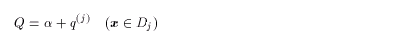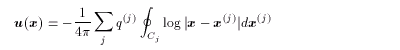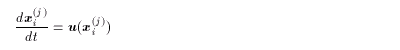3. Numerical Methods
3.1 Numerical Procedure of the Contour Dynamics Model
3.2 Other Details
3.3 Integral Calculation

### 3.1 Numerical Procedure of the Contour Dynamics Model

< Summary of the theory (section 2.2) >

1. The vorticity distribution is assumed to be(3.1)
for regions Dj ( j=1,2, ... ,J ) ( Dj1Ç Dj2 = j ) on an infinite plane.

2. The velocity at an arbitrary point on the plane is calculated using the points x(j) on each contour Cj as(3.2)

< Procedure to calculate the time evolution of the vorticity distribution >

1. Calculate the velocities of the nodes { xi(j) } on the contours by using (3.2):(3.3)

2. Integrate (3.3) to know the positions of the contours at the next time t + Δt .

3. Repeat these processes to obtain the time evolution.

<<index <previous next>